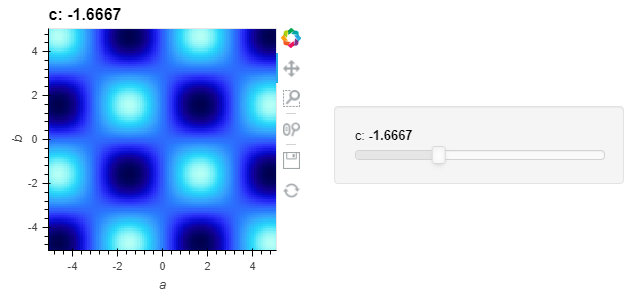# PointerXY/Text with dynamic image source

Hi,
Let’s say I have a 3D array that I want to display different slices of (that part is fairly standard):

``````import numpy as np
import xarray as xr
import holoviews as hv
hv.extension('bokeh')

ls = np.linspace(-5, 5, 100)
xx, yy,zz = np.meshgrid(ls, ls, ls)
img_np = np.sin(xx) * np.cos(yy+zz)
img_xr = xr.DataArray(data=img_np, dims=['a','b','c'], coords=[ls, ls, ls])

def plot_c_slice(c):
return hv.Image(img_xr.sel(c=c))
dmap = hv.DynamicMap(plot_c_slice, kdims=['c']).redim.values(c=ls)
dmap
``````I’m interested in adding a PointerXY / crosshair (both are practically the same example, BTW).

That example (in the links above) is for the simple case of a ‘static’ image (2D array), yet as I’m viewing different slices I need to get the dynamic parameter (‘c’ in the example above) into cross_hair_info function. E.g., let’s say I’m interested in text displaying mean(a,b,c), where a,b are the 2D spatial coordinates of the image, and c is the slice.

How would I achieve that?

I have to admit that although I’ve looked at some of the references related to this topic, I’m feeling a bit lost…
httpXXX://holoviews.org/user_guide/Building_Composite_Objects.html
httpXXX://holoviews.org/user_guide/Live_Data.html
httpXXX://holoviews.org/user_guide/Responding_to_Events.html
httpXXX://github.com/holoviz/holoviews/issues/1184

Thanks.

Hi, a solution is to create a param.Parameterized class in wich you put the stream , the cross_hair_info and you create an attribute c and a function that will return your plot.

Here is the code :

``````from holoviews import streams
import holoviews as hv
import panel as pn
import param
import numpy as np
import xarray as xr
hv.extension('bokeh')

class Example(param.Parameterized):
ls = np.linspace(-5, 5, 100)
c = param.Selector(default=ls,objects=ls)
xx, yy,zz = np.meshgrid(ls, ls, ls)
img_np = np.sin(xx) * np.cos(yy+zz)
img_xr = xr.DataArray(data=img_np, dims=['a','b','c'], coords=[ls, ls, ls])
img = hv.Image(img_xr.sel(c=ls))
pointer = streams.PointerXY(x=0, y=0, source=img)

def cross_hair_info(self,x, y):
text = hv.Text(x+0.05, y, '%.3f'% np.mean([x,y,self.c]), halign='left', valign='bottom')
return hv.HLine(y) * hv.VLine(x) * text

@param.depends('c')
def view(self):
self.img = hv.Image(self.img_xr.sel(c=self.c))
pointer = streams.PointerXY(x=0, y=0, source=self.img)
return self.img*hv.DynamicMap(self.cross_hair_info, streams=[pointer])

ex = Example()

pn.Row(ex.view,pn.Param(ex.param,widgets={'c':pn.widgets.DiscreteSlider}))

``````

I don’t know if it’s the best solution maybe someone have a better one. But It can be a great start for you.

Thanks AurelienSciarra.
Using this param module seems like an elegant solution, I’ll have to do some reading on that.

For future generations, those seem to be good starting places:
https://panel.holoviz.org/user_guide/Param.html
http://holoviews.org/user_guide/Dashboards.html